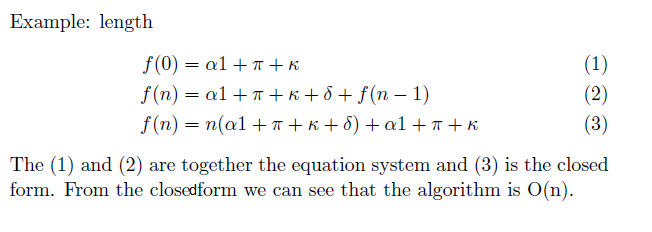# Analysing of F# Programs

## Sunday, June 06, 2010 »programming, uni

This class covers in fact labour work. The aim of this labour was to create an extensible framework to establish a highly customizable and flexible scanner for the F# functional programming language. In the frame of the labour my part was the resource analysis of F# programs to analyzing the cost of functional codes. The main difficulties with this approach that the complex [or even not so complex] recursive functions generate multi variables functions that - we know - it's not simple to solve. There is no general concept to solve so different type of multi variables functions. One promising attemption was to convert such functions to closed form and analyzing these formulas. Near the concept,the technology solution needed to be figured out and integrated it into our FSharp Tool. The imagination was to integrate it in the tool where the resource of currently implemented source code would be shown automatically on some IDE panel.

One conception was to examine the Theorema System to solving the recursive equations. The Theorema is a software system for computer supported mathematical theorem proving and theory exploration. Unfortunately just before I began the cognition with the system, the whole project was frozen. The beginning of examination of connection between .NET and Theorema remained also in theoritical phase (SimpleLink, JLink, NETLink). Some other points where I [would] have started...

Mathematica Tutorial - Solving Recurrence Equations
Tom Leighton - Notes on Better Master Theorems for Divide-and-Conquer Recurrences
William L. Harrison, Ph.D - A Simple Semantics for Polymorphic Recursion
https://plato.stanford.edu/entries/recursive-functions/
Unknown - Example for analysing recursive methods
Frdric Gava - BSP Functional Programming: Examples of a Cost Based Methodology
Pedro B. Vasconcelos? and Kevin Hammond - Inferring Cost Equations for Recursive, Polymorphic and Higher-Order Functional Programs
Bent E. Petersen - Linear Recurrence Relations in Maple
Richard Bird, Jeremy Gibbons and Geraint Jones - Program optimisation, naturally
Pedro Baltazar Vasconcelos - Space Cost Analysis Using Sized Types
http://math.furman.edu/~dcs/book/c1pdf/sec14.pdf[Analysis of F# Programs] [Analysis of F# Codes]